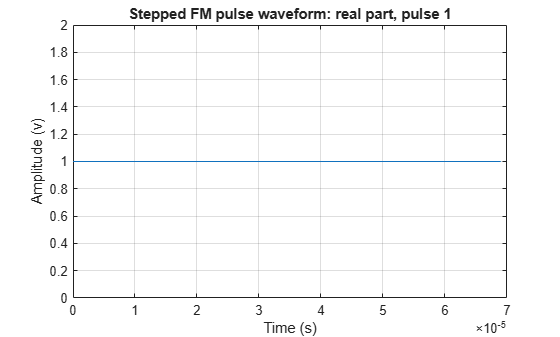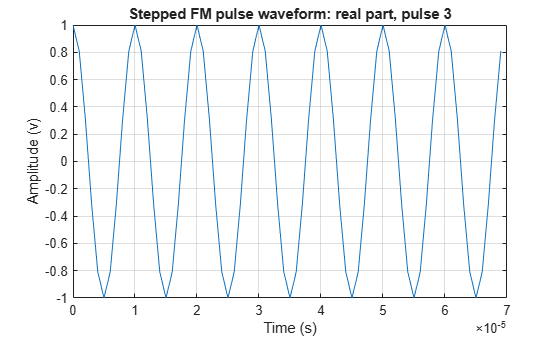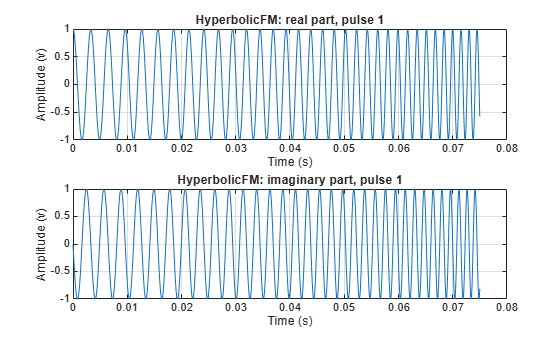# pulseWaveformLibrary

Create library of pulse waveforms

## Description

The `pulseWaveformLibrary` System object™ creates a library of pulse waveforms. The waveforms in the library can be of different types or be of the same type with different parameters. You can use this library to transmit different kinds of pulses during a simulation.

To make a waveform library

1. Create the `pulseWaveformLibrary` object and set its properties.

2. Call the object with arguments, as if it were a function.

## Creation

### Syntax

``pulselib = pulseWaveformLibrary``
``pulselib = pulseWaveformLibrary(Name,Value)``

### Description

````pulselib = pulseWaveformLibrary` System object creates a library of pulse waveforms, `pulselib`, with default property values. The default consists of a rectangular waveform and a linear FM waveform.```

example

````pulselib = pulseWaveformLibrary(Name,Value)` creates a pulse waveform library with each property `Name` set to a specified `Value`. You can specify additional name-value pair arguments in any order as (`Name1`,`Value1`,...,`NameN`,`ValueN`). Enclose each property name in single quotes.Example: ```pulselib = pulseWaveformLibrary('SampleRate',1e9,'WaveformSpecification',{{'Rectangular','PRF',1e4,'PulseWidth',100e-6},{'SteppedFM','PRF',1e4}})``` creates a library containing one rectangular waveform and one stepped-FM waveform, both sampled at 1 GHz.```

## Properties

expand all

Unless otherwise indicated, properties are nontunable, which means you cannot change their values after calling the object. Objects lock when you call them, and the `release` function unlocks them.

If a property is tunable, you can change its value at any time.

Waveform sample rate, specified as a positive scalar. All waveforms have the same sample rate. Units are in hertz.

Example: `100e3`

Data Types: `double`

Pulse waveforms, specified as a cell array. Each cell of the array contains the specification of one waveform.

`{{Waveform 1 Specification},{Waveform 2 Specification},{Waveform 3 Specification}, ...}`
Each waveform specification is also a cell array containing the parameters of the waveform. The entries in a specification cell are the pulse identifier and a set of name-value pairs specific to that waveform.
`{PulseIdentifier,Name1,Value1,Name2,Value2, ...}`
This System object supports four built-in waveforms and also lets you specify custom waveforms. For the built-in waveforms, the waveform specifier consists of a waveform identifier followed by several name-value pairs setting the properties of the waveform. For the custom waveforms, the waveform specifier consists of a handle to a user-define waveform function and the functions input arguments.

Waveform Types

 Waveform type Waveform identifier Waveform arguments Linear FM `'LinearFM'` Linear FM Waveform Arguments Phase coded `'PhaseCoded'` Phase-Coded Waveform Arguments Rectangular `'Rectangular'` Rectangular Waveform Arguments Stepped FM `'SteppedFM'` Stepped FM Waveform Arguments Custom Function handle Custom Waveform Arguments

Example: `{{'Rectangular','PRF',10e3,'PulseWidth',100e-6},{'Rectangular','PRF',100e3,'PulseWidth',20e-6}}`

Data Types: `cell`

### Linear FM Waveform Arguments

Specify optional comma-separated pairs of `Name,Value` arguments. `Name` is the argument name and `Value` is the corresponding value. `Name` must appear inside quotes. You can specify several name and value pair arguments in any order as `Name1,Value1,...,NameN,ValueN`.

Example: ```{'LinearFM','PRF',1e4,'PulseWidth',50e-6,'SweepBandwidth',1e5,... 'SweepDirection','Up','SweepInterval','Positive'}```

Pulse repetition frequency (PRF), specified as a positive scalar. Units are in hertz. See Pulse Repetition Frequency Restrictions for restrictions on the PRF.

Example: `20e3`

Data Types: `double`

Pulse duration, specified as a positive scalar. Units are in seconds. You cannot specify both `PulseWidth` and `DutyCycle`.

Example: `100e-6`

Data Types: `double`

Pulse duty cycle, specified as a positive scalar greater than zero and less than or equal to one. You cannot specify both `PulseWidth` and `DutyCycle`.

Example: `0.7`

Data Types: `double`

Bandwidth of the FM sweep, specified as a positive scalar. Units are in hertz.

Example: `100e3`

Data Types: `double`

Direction of the FM sweep, specified as `'Up'` or `'Down'`. `'Up'` corresponds to increasing frequency. `'Down'` corresponds to decreasing frequency.

Data Types: `char`

FM sweep interval, specified as `'Positive'` or `'Symmetric'`. If you set this property value to `'Positive'`, the waveform sweeps the interval between 0 and B, where B is the `SweepBandwidth` argument value. If you set this property value to `'Symmetric'`, the waveform sweeps the interval between –B/2 and B/2.

Example: `'Symmetric'`

Data Types: `char`

Envelope function, specified as `'Rectangular'` or `'Gaussian'`.

Example: `'Gaussian'`

Data Types: `char`

Frequency offset of pulse, specified as a scalar. The frequency offset shifts the frequency of the generated pulse waveform. Units are in hertz.

Example: `100e3`

Data Types: `double`

### Phase-Coded Waveform Arguments

Specify optional comma-separated pairs of `Name,Value` arguments. `Name` is the argument name and `Value` is the corresponding value. `Name` must appear inside quotes. You can specify several name and value pair arguments in any order as `Name1,Value1,...,NameN,ValueN`.

Example: ```{'PhaseCoded','PRF',1e4,'Code','Zadoff-Chu', 'SequenceIndex',3,'ChipWidth',5e-6,'NumChips',8}```

Pulse repetition frequency (PRF), specified as a positive scalar. Units are in hertz. See Pulse Repetition Frequency Restrictions for restrictions on the PRF.

Example: `20e3`

Data Types: `double`

Type of phase modulation code, specified as `'Frank'`, `'P1'`, `'P2'`, `'Px'`, `'Zadoff-Chu'`, `'P3'`, `'P4'`, or `'Barker'`.

Example: `'P1'`

Data Types: `char`

Sequence index used for the `Zadoff-Chu` code, specified as a positive integer. The value of `SequenceIndex` must be relatively prime to the value of `NumChips`.

Example: `3`

#### Dependencies

To enable this name-value pair, set the `Code` property to `'Zadoff-Chu'`.

Data Types: `double`

Chip duration, specified as a positive scalar. Units are in seconds. See Chip Restrictions for restrictions on chip sizes.

Example: `30e-3`

Data Types: `double`

Number of chips in waveform, specified as a positive integer. See Chip Restrictions for restrictions on chip sizes.

Example: `3`

Data Types: `double`

Frequency offset of pulse, specified as a scalar. The frequency offset shifts the frequency of the generated pulse waveform. Units are in hertz.

Example: `100e3`

Data Types: `double`

### Rectangular Waveform Arguments

Specify optional comma-separated pairs of `Name,Value` arguments. `Name` is the argument name and `Value` is the corresponding value. `Name` must appear inside quotes. You can specify several name and value pair arguments in any order as `Name1,Value1,...,NameN,ValueN`.

Example: `{'Rectangular','PRF',10e3,'PulseWidth',100e-6}`

Pulse repetition frequency (PRF), specified as a positive scalar. Units are in hertz. See Pulse Repetition Frequency Restrictions for restrictions on the PRF.

Example: `20e3`

Data Types: `double`

Pulse duration, specified as a positive scalar. Units are in seconds. You cannot specify both `PulseWidth` and `DutyCycle`.

Example: `100e-6`

Data Types: `double`

Pulse duty cycle, specified as a positive scalar greater than zero and less than or equal to one. You cannot specify both `PulseWidth` and `DutyCycle`.

Example: `0.7`

Data Types: `double`

Frequency offset of pulse, specified as a scalar. The frequency offset shifts the frequency of the generated pulse waveform. Units are in hertz.

Example: `100e3`

Data Types: `double`

### Stepped FM Waveform Arguments

Specify optional comma-separated pairs of `Name,Value` arguments. `Name` is the argument name and `Value` is the corresponding value. `Name` must appear inside quotes. You can specify several name and value pair arguments in any order as `Name1,Value1,...,NameN,ValueN`.

Example: `{'SteppedFM','PRF',10e-4}`

Pulse repetition frequency (PRF), specified as a positive scalar. Units are in hertz. See Pulse Repetition Frequency Restrictions for restrictions on the PRF.

Example: `20e3`

Data Types: `double`

Pulse duration, specified as a positive scalar. Units are in seconds. You cannot specify both `PulseWidth` and `DutyCycle`.

Example: `100e-6`

Data Types: `double`

Pulse duty cycle, specified as a positive scalar greater than zero and less than or equal to one. You cannot specify both `PulseWidth` and `DutyCycle`.

Example: `0.7`

Data Types: `double`

Number of frequency steps in waveform, specified as a positive integer.

Example: `3`

Data Types: `double`

Linear frequency step size, specified as a positive scalar.

Example: `100.0`

Data Types: `double`

Frequency offset of pulse, specified as a scalar. The frequency offset shifts the frequency of the generated pulse waveform. Units are in hertz.

Example: `100e3`

Data Types: `double`

### Custom Waveform Arguments

You can create a custom waveform from a user-defined function. The first input argument of the function must be the sample rate. For example, specify a hyperbolic waveform function,

`function wav = HyperbolicFM(fs,prf,pw,freq,bw,fcent),`
where `fs` is the sample rate and `prf`, `pw`, `freq`, `bw`, and `fcent` are other waveform arguments. The function must have at least one output argument, `wav`, to return the samples of each pulse. This output must be a column vector. There can be other outputs returned following the waveform samples.

Then, create a waveform specification using a function handle instead of the waveform identifier. The first cell in the waveform specification must be a function handle. The remaining cells contain all function input arguments except the sample rate. Specify all input arguments in the order they are passed into the function.

`waveformspec = {@HyperbolicFM,prf,pw,freq,bw,fcent}`
See Add Custom Waveform to Pulse Waveform Library for an example that uses a custom waveform.

## Usage

### Syntax

``waveform = pulselib(idx)``

### Description

example

````waveform = pulselib(idx)` returns samples of a waveform, `waveform`, specified by its index, `idx`, in the library.```

### Input Arguments

expand all

Index of the waveform in the waveform library, specified as a positive integer.

Example: `2`

Data Types: `double`

### Output Arguments

expand all

Waveform samples, returned as a complex-valued vector.

Data Types: `double`
Complex Number Support: Yes

## Object Functions

To use an object function, specify the System object as the first input argument. For example, to release system resources of a System object named `obj`, use this syntax:

`release(obj)`

expand all

 `getMatchedFilter` Matched filter coefficients for pulse waveform `plot` Plot waveform from waveform library
 `step` Run System object algorithm `release` Release resources and allow changes to System object property values and input characteristics `reset` Reset internal states of System object

## Examples

collapse all

Construct a waveform library consisting of three waveforms. The library contains a rectangular, a linear FM, and a phase-coded waveform. Then, obtain and plot the real and imaginary parts of the phase-coded waveform.

```waveform1 = {'Rectangular','PRF',1e4,'PulseWidth', 50e-6}; waveform2 = {'LinearFM','PRF',1e4,'PulseWidth',50e-6, ... 'SweepBandwidth',1e5,'SweepDirection','Up',... 'SweepInterval', 'Positive'}; waveform3 = {'PhaseCoded','PRF',1e4,'Code','Zadoff-Chu', ... 'SequenceIndex',3,'ChipWidth',5e-6,'NumChips',8}; fs = 1e6; wavlib = pulseWaveformLibrary('SampleRate',fs, ... 'WaveformSpecification',{waveform1,waveform2,waveform3});```

Extract the waveform from the library.

`wav3 = wavlib(3);`

Plot the waveform using the `plot` method.

`plot(wavlib,3,'PlotType','complex')`Construct a waveform library consisting of three waveforms. The library contains one rectangular, one linear FM, and one stepped-FM waveforms. Then, plot the real parts of the first three pulses of the stepped-fm waveform.

```waveform1 = {'Rectangular','PRF',1e4,'PulseWidth',70e-6}; waveform2 = {'LinearFM','PRF',1e4,'PulseWidth',70e-6, ... 'SweepBandwidth',1e5,'SweepDirection','Up', ... 'SweepInterval', 'Positive'}; waveform3 = {'SteppedFM','PRF',1e4,'PulseWidth', 70e-6,'NumSteps',5, ... 'FrequencyStep',50000,'FrequencyOffset',0}; fs = 1e6; wavlib = pulseWaveformLibrary('SampleRate',fs, ... 'WaveformSpecification',{waveform1,waveform2,waveform3});```

Plot the first three pulses of the waveform using the `plot` method.

`plot(wavlib,3,'PulseIdx',1)``plot(wavlib,3,'PulseIdx',2)``plot(wavlib,3,'PulseIdx',3)`This example shows how to put two waveforms into a waveform library and how to extract and plot their matched filter coefficients.

Create a pulse library consisting of a rectangular and a linear FM waveform.

```waveform1 = {'Rectangular','PRF',10e3 'PulseWidth',50e-6}; waveform2 = {'LinearFM','PRF',10e3,'PulseWidth',50e-6,'SweepBandwidth',1e5, ... 'SweepDirection','Up','SweepInterval', 'Positive'}; pulsesib = pulseWaveformLibrary('SampleRate',1e6,... 'WaveformSpecification',{waveform1,waveform2});```

Retrieve the matched filter coefficients for each waveform and plot their real parts.

```coeff1 = getMatchedFilter(pulsesib,1,1); subplot(2,1,1) stem(real(coeff1)) title('Matched filter coefficients, real part') coeff2 = getMatchedFilter(pulsesib,2,1); subplot(2,1,2) stem(real(coeff2)) title('Matched filter coefficients, real part')```Define a custom hyperbolic FM waveform and add it to a p`ulseWaveformLibrary` System object together with a linear FM waveform. Plot the hyperbolic waveform.

Specify the hyperbolic FM waveform parameters. The pulse width is 75 ms and the pulse repetition interval is 100 ms. The center frequency is 500 Hz and the bandwidth is 400 Hz.

```fs = 50e3; pri = 0.1; prf = 1/pri; pw = 0.075; bw = 400.0; fcent = 500.0;```

Create a pulse waveform library consisting of a hyperbolic FM waveform and a linear FM waveform.

```pulselib = pulseWaveformLibrary('SampleRate',fs, ... 'WaveformSpecification',{{@HyperbolicFM,prf,pw,bw,fcent}, ... {'LinearFM','PRF',prf,'PulseWidth',pw, ... 'SweepBandwidth',bw,'SweepDirection','Up',... 'SweepInterval','Positive'}});```

Plot the complex hyperbolic FM waveform.

`plot(pulselib,1,'PlotType','complex')`Define the Hyperbolic FM waveform function.

```function y = HyperbolicFM(fs,prf,pw,bw,fcent) pri = 1/prf; t = [0:1/fs:pri]'; idx = find(t <= pw); fl = fcent - bw/2; fh = fcent + bw/2; y = zeros(size(t)); arg = 2*pi*fl*fh/bw*pw*log(1.0 - bw*t(idx)/fh/pw); y(idx) = exp(1i*arg); end```

expand all

## Extended Capabilities

Introduced in R2021a